# Correlation Analysis Sample Assignment

## Abstract

As we know that Money is a very important requirement for the economy and surviving the life. So, it depends on the different factors of the economy. In this dataset we have given some attributes. These attributes are INF, RGDP, M2, XR, IR, Year. Out of this given attributes Money depends on the RGDP, IF, XR, IR. In this assignment data is collected from the Year 1980 to 2015. The graph of the each attribute of the dataset is given as follows: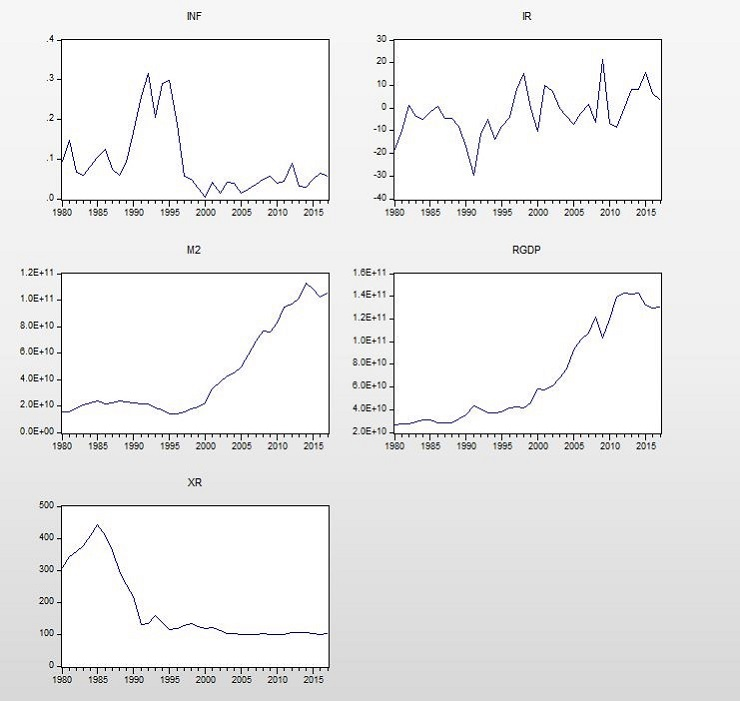In these graphs we can see that the pattern of the RGDP and money attribute is almost similar. In the given dataset Money is represented by the M2. In this we have observed the fluctuation between the demand of money. In the graph we can see that in the year 1995 money has started decreasing but after that it is increasing. In the initial year it has decreased but after that it has started to increase. Same pattern is with the other attributes but there is slightly difference the graph and trend in the attributes. The XR attribute has decreasing trend in initial years but after that it has started to increase.

## Correlation Analysis

The table which is given below represents the correlation between the variables: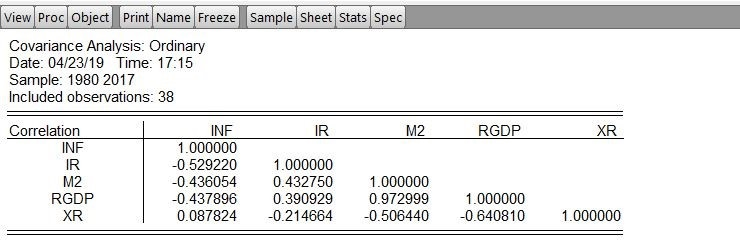In this correlation between the all variables shows that positive values of 1. It represents that strong positive correlation. The positive value of money with variable RGDP is 0.97 which shows the strong positive correlation with this attribute. While the demand of money with exchange rate is negative. Hence, we can say that it has positive impact on demand of money. The correlation value of money is negative with the inflation.

Model Output:

In this dataset we have implemented the least squares. In this model we have made the money has dependent variables while we have taken the exchange rate as independent variable on which our money is dependent. In this we got to know that the results of the implementation of model is as follows: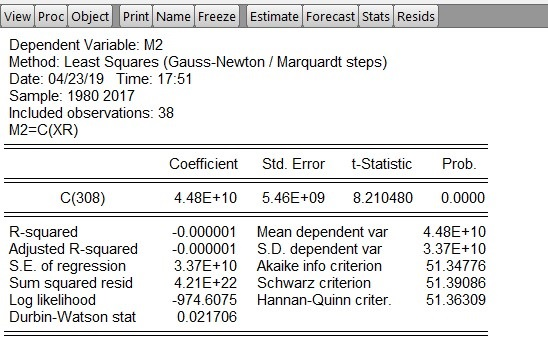This represent the residual graph of the dataset. In this graph we can see the three lines.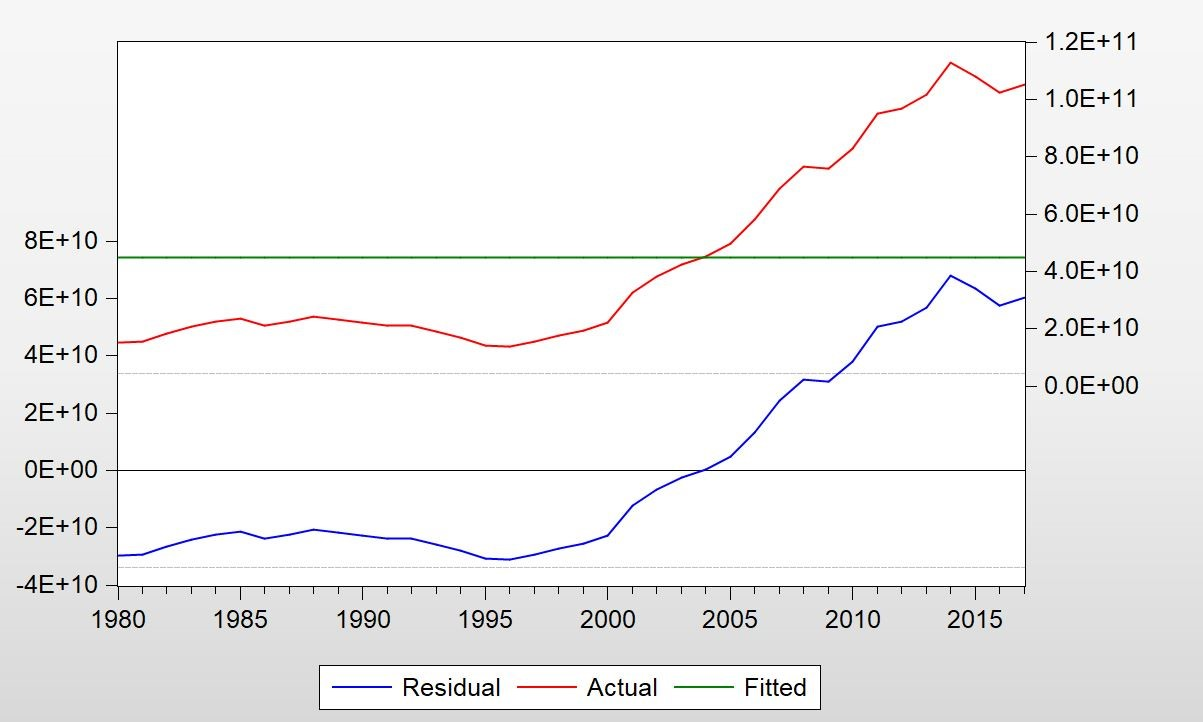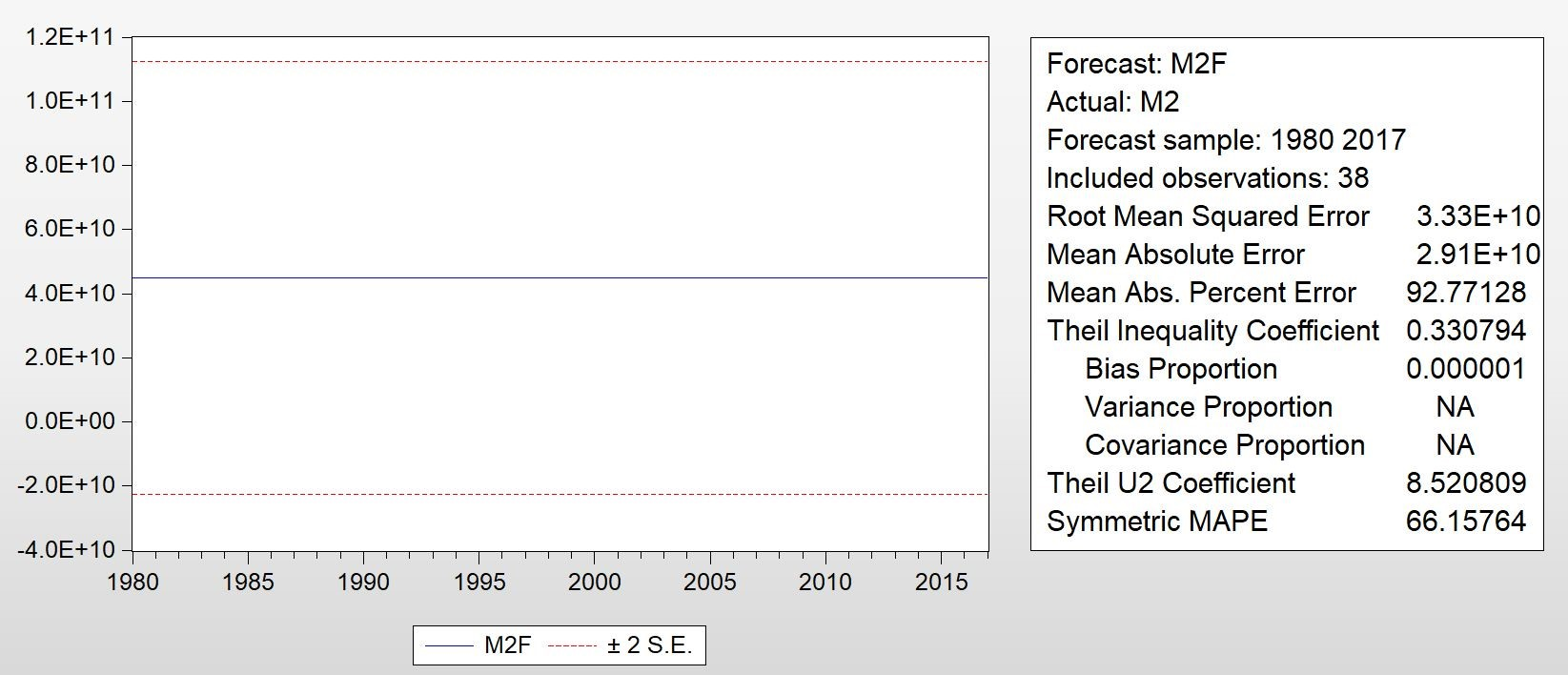## Descriptive Statistics

Descriptive statistics for all the variables are shown below: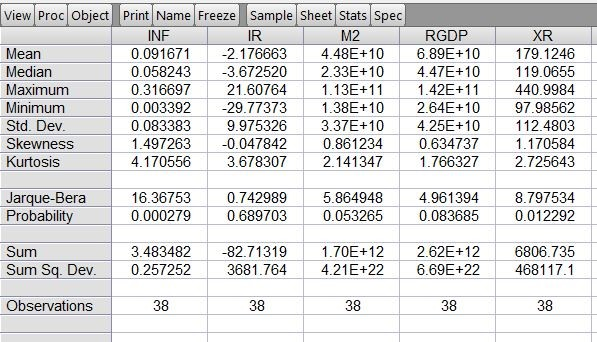The descriptive statistics of the dataset is given above. In this we can see that Mean of money is 4.48, maximum value is 1.13 and minimum value is 1.38. There is very less variation in demand of money.

The mean of RGDP is 6.89, maximum value is 1.42 and minimum value is 2.64.

The mean of inflation is 0.09, maximum value is 0.31 and minimum value is 0.003.

In this we have shown the different statistical results of the data set which includes all the statistical values of the attributes.